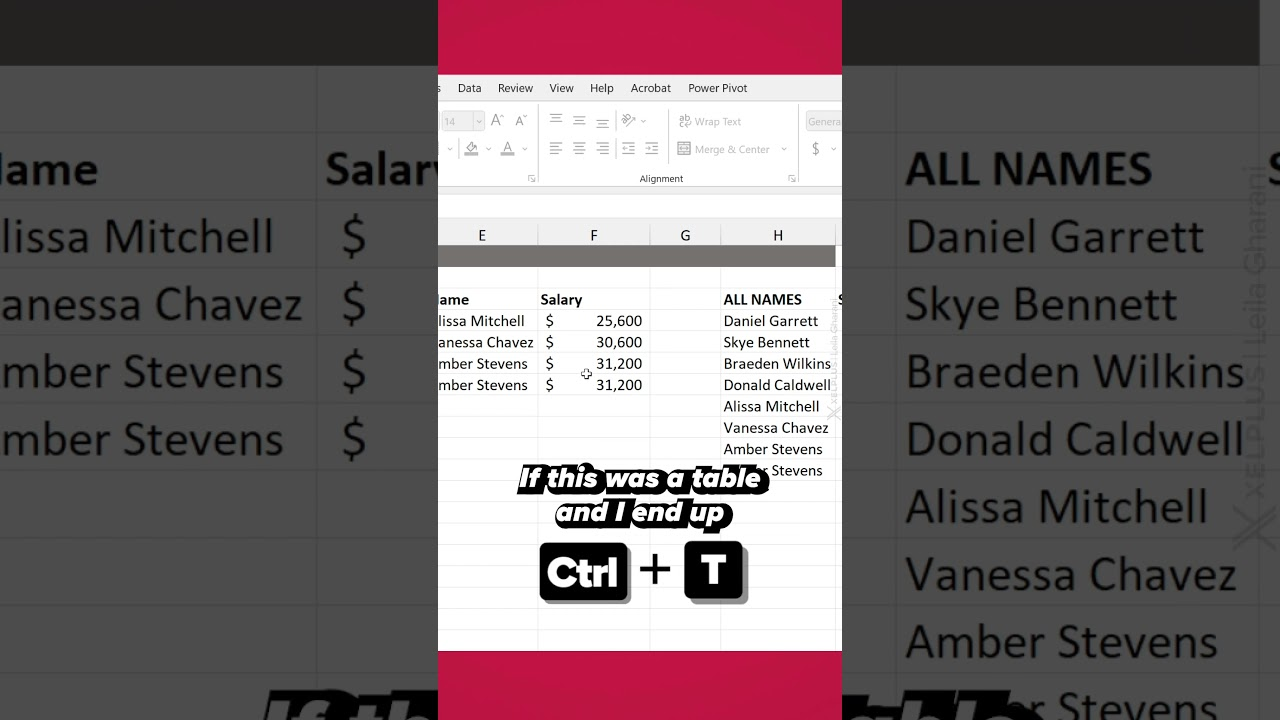en | deExcel
May 12, 2023 10:00 AM

# How to Use VSTACK and HSTACK Function in Excel

by HubSite 365 about Leila Gharani [MVP]
Pro User

Excel

## With these power functions you can append your data together. VSTACK dynamically combines arrays

You’re going to love the new VSTACK & HSTACK functions in Microsoft Excel! With these power functions you can append your data together. VSTACK dynamically combines arrays into a single vertical array while HSTACK combines them horizontally. In this video I’ll show you how it works.

Requires Microsoft 365. The TAKE function will not be available in Excel 2021 and earlier versions.

In Excel, VSTACK and HSTACK are not built-in functions. Instead, you can use the CONCATENATE function or the “&” operator to join cells horizontally, and the “Ctrl” + “Shift” + “Enter” array formula to join cells vertically. However, in this context, I’ll explain how to achieve similar results to VSTACK and HSTACK using Excel’s built-in functions and shortcuts.

## HSTACK (Horizontal Stack)

To stack cells horizontally, you can use the CONCATENATE function or the “&” operator.

Using CONCATENATE:

=CONCATENATE(A1, A2, A3)

Using “&” operator:

=A1 & A2 & A3

Both of these methods will horizontally stack the contents of cells A1, A2, and A3.

## VSTACK (Vertical Stack)

To stack cells vertically, you need to create an array formula using “Ctrl” + “Shift” + “Enter”. Here’s how:

a. Click on the cell where you want the vertical stack to begin.

b. Type the following formula, replacing A1:A3 with your range of cells:

=TRANSPOSE(A1:A3)

c. Press “Ctrl” + “Shift” + “Enter” simultaneously. Excel will add curly braces {} around the formula, indicating it’s an array formula.

The contents of cells A1, A2, and A3 will now be stacked vertically in the column where you entered the formula.

Note: The TRANSPOSE function changes the orientation of the selected range, so it effectively stacks cells vertically when used in a single column.

HubSite 365 Apps